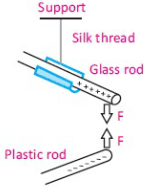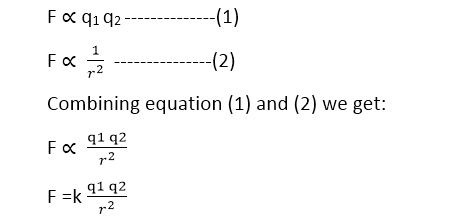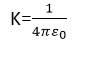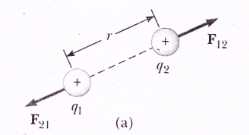Electricity & Megnetism

# Coulomb’s law formula with examples and problems

## What is Coulomb’s law?

“Coulomb’s law states that force of attraction or repulsion between two electric charges is directly proportional to their magnitudes and inversely proportional to the square of the distance between them”.Coulomb’s law examples are given below.

Charles Agustin Coulomb (1736-1806) measured electrical attractions and repulsion quantitatively and deduced the law that governs them.

This figure resembles the figure given below:If a and b are charged, the electric force on a tends to twist the suspension fiber. Coulomb canceled out this twisting effect by turning the suspension head through the angle θ needed to keep the two charges at a particular separation. The angle θ is then a relative measure of the electric force acting on charge a. The device of the above figure is a torsion balance, a similar arrangement was used later by Cavendish to measure gravitational attractions.

## Coulomb’s Law formula & its DerivationThis relation is called the coulomb’s law. Here F is called the magnitude of the mutual force that acts on each of the two charges a and b, qand q2 are relative measures of the charges on spheres a and b, and r is the distance between their centers. The force on each charge is due to the other acts along the line connecting the charges. The two forces point in opposite directions but have equal magnitudes, even though the charges may be different. The two forces point in opposite directions but have equal magnitudes, even though the charges may be different.

### Coulomb’s constant

To turn the above proportionality into an equation, let us introduce a constant of proportionality, which we represent for now as k. Its value depends upon the system of units and the medium between the charges. For free space and in the system international ‘k’ is expressed as:### The permittivity of free space

Where ε0 is the permittivity of free space and its value is 8.85 × 10-12C-2N-1m-2.

we thus obtain, for the force between the charges. Coulomb’s law generally holds only for charged objects whose sizes are much smaller than the distance between them. We often say that it holds only for point charges.

Our belief in coulomb’s law does not rest quantitatively on coulomb’s experiments. Torsion balance measurements are difficult to make to an accuracy of better than a few percent.

Coulomb’s law resembles Newton,s inverse square law of gravitation, which was already more than 100 years old at the time of Coulomb’s experiments. Both are inverse-square laws, and the charge q plays the same role in Coulomb’s law that the mass m plays in Newton’s law of gravitation.

One difference between the two laws is that gravitational forces, as far as we know, are always attractive, while electrostatic forces can be repulsive or attractive, depending on whether the two charges have the same or opposite signs.

There is another important difference between the two laws. In using the law of gravitation, we were able to define mass from Newton’s second law, and then by applying the law of gravitation to know masses we could determine the constant G.in using the Electrostatic force law, we take the reverse approach; we define the constant k to have a particular value, and we then use Coulomb’s law to determine the basic unit of electric charge as the quantity of charge that produces a standard unit of force.

For example, consider the force between two equal charges of magnitude q. We could adjust q until the force has a particular value, say 1 N for separation of r=1m, and define the resulting q as the basic unit of charge. It is, however, more precise to measure the magnetic force between two wires carrying equal currents, and therefore the fundamental SI electrical unit is the unit of current, from which the unit of charge is derived. The operational procedure for defining the SI unit of current is called the ampere.

## Coulomb’s law in vector form

Coulomb’s force is a mutual force, it means that if charge ‘q1‘ exerts a force on charge ‘q2‘ then q2 also exerts an equal and opposite force on q1.If charge qexerts an electrostatic force “F12 charge q2 and q2 exert electrical force F21 on charge q1.

If r12 represents the direction of force from charge qto charge qand r21 is the unit vector that represents the direction of force from charge  qto q1, then:According to newton’s 3rd law:

F21=-F12

This expression shows that the Coulomb force is a mutual force.

### Significance of vector form of coulomb law

The vector form of coulomb law has critical importance when there is an assembly of point charges. In this case, the resultant force on any one of the charges is the vector sum of the forces due to each of the other forces. This is called the principle of superposition.

### Coulomb’s law examples in physics

Now!

1.Muhammad Bilal Dayo says: